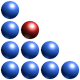# Cellular Automatons and Complexity

From the Wikipedia: A cellular automaton is a discrete model studied in computability theorymathematicsphysicscomplexity sciencetheoretical biology and microstructure modelling. It consists of a regular grid of cells, each in one of a finite number of states, such as “On” and “Off” (in contrast to a coupled map lattice). The grid can be in any finite number of dimensions.

For each cell, a set of cells called its neighbourhood (usually including the cell itself) is defined relative to the specified cell. For example, the neighbourhood of a cell might be defined as the set of cells a distance of 2 or less from the cell. An initial state (time t=0) is selected by assigning a state for each cell. A new generation is created (advancing t by 1), according to some fixed rule (generally, a mathematical function) that determines the new state of each cell in terms of the current state of the cell and the states of the cells in its neighbourhood. For example, the rule might be that the cell is “On” in the next generation if exactly two of the cells in the neighbourhood are “On” in the current generation, otherwise the cell is “Off” in the next generation. Typically, the rule for updating the state of cells is the same for each cell and does not change over time, and is applied to the whole grid simultaneously, though exceptions are known.

We have measured the complexity and extracted the complexity map of a few cellular automatons which may be found here and are illustrated in the image below:

While  humans are good at recognising patterns and structure, rapid classification of patterns in terms of their complexity is not easy. For example, which is more complex in the above figure, Rule 250 or Rule 190? The answer is below.

Rule 30

Rule 54

Rule 62

Rule 90

Rule 190

Rule 250

It appears that Rule 250 Automaton is the most complex of all (C = 186.25) , while the one with the lowest complexity is Rule 90 (C = 64.31). Not very intuitive, is it?

Intuition is given only to him who has undergone long preparation to receive it (L. Pasteur).